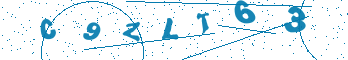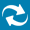# Time Calculations in VHDL

## Introduction

VHDL uses quite unique concept of time that may cause problems for some users when they attempt non-trivial time computations. This document explains how to use both built-in time data type facilities and functions from aldec_tools package that provide additional, beneficial functionality.

## The "time" type

VHDL defines built-in time type like this:

```	type time is range -2147483647 to 2147483647
units
fs;
ps = 1000 fs;
ns = 1000 ps;
us = 1000 ns;
ms = 1000 us;
sec = 1000 ms;
min = 60 sec;
hr = 60 min;
end units;
```

It is a textbook example of physical type with primary unit: femtoseconds and secondary units up to an hour. Please note that range of values of the type is described using 32-bit signed integer, not real type. It means that the granularity of time in VHDL cannot be finer than 1 fs: decimal fractions of time can only happen if the secondary time units are used.

The question we should ask is: can we use 32-bit numbers to store time values in the simulator, no matter what units are used? Let's see:

• An hour has 3.6x1018 femtoseconds.

• The maximal value that can be expressed in 32-bit signed integers is 231-1 = 2147483647 = 2.15x109.

It means that 32 bits is not enough to cover all predefined time units. We would run out of range after reaching 2.15 microseconds! That is the main reason why simulators are not using 32-bit integers to represent time.

If not 32-bit, what else? Let's check the next popular size of integers = 64 bit:

• The maximal value that can be expressed in 64-bit signed integers is 263-1 = 9223372036854775807 = 9.22x1018.

• When divided by 3.6x1018 it gives 2.5620477880152.

It means that 64-bit signed integers can cover time values within 1 femtosecond to over 2.56 hour range, which seems reasonable for majority of applications. That's why VHDL simulators typically use 64-bit time representation.

If we know that the smallest delay needed during simulation is 100 picoseconds and we will be simulating until we reach 100 microseconds, it means that simulator will be using time values within 105 to 1011 range, i.e. there will always be 5 dangling zeroes at the end of time values. Not nice, and definitely not economical! To solve this little issue, simulators typically use the concept of simulation resolution: the smallest time value that simulator is obliged to recognize during given simulation session. By specifying simulation resolution larger than 1 femtosecond we can speed up the simulation with one penalty: any time value that is smaller than resolution will be rounded down to zero.

## Time conversions

When writing testbenches, VHDL users may be forced to convert between time and abstract numeric types (integer and real). The simplest conversion is from integer to time:

```	-- Time_value := Int_value * Time_unit;
timeout := intmax * 1 ns;
```

Conversion from real to time requires additional typecast (type conversion):

```	-- Time_value := integer(Real_value) * Time_unit;
timeout := integer(realmax) * 1 ns;>
```

(Please note that if the real value has fractional part that we want to preserve, we have to rescale the value and adjust time unit appropriately.)

Conversions in the opposite direction are more difficult because they require division operation. In many cases this operation can be executed without any problems, but quite unexpectedly it can trigger division by zero or overflow problems.

The first stage of converting time value to abstract value is unit stripping, which requires division of time value by time unit. This operation yields integer value that can be typecasted to real and rescaled if needed:

```	Int_pico := curr_time / 1 ns * 1000;
Real_pico := real(curr_time / 1 ns) * 1000.0;>
```

What happens if we have simulation resolution set to a value larger than the divisor used in the conversion? Of course the divisor will be rounded down to zero, which will trigger "division by zero" error and termination of simulation.

What happens if the time value (in the divisor units) is greater than 231-1 (e.g. curr_time = 3 sec) in our sample code above? Since the division result is out of range that integers can handle, we will experience overflow. It does not necessarily mean termination of simulation, but incorrect conversion (most significant bit of the division result treated as the sign bit of the integer value).

## aldec_tools package

In the most recent releases of simulators ALDEC provides aldec_tools package in the aldec library. Among other features, it contains three useful functions:

```	function to_real ( time_val : in time ) return real;
function to_time ( real_val : in real ) return time;
function get_resolution return real;>
```

The get_resolution function without parameters returns real number representing simulation resolution expressed in seconds. If your simulator has resolution set to 1 picosecond, the function will return 10-12.

The to_real function returns real value of time parameter scaled to current resolution unit. If simulation resolution is 10 picoseconds, to_real(1 ns) returns 100.0 .

The to_time function will return time value of its real argument, treating the argument as the number of simulation resolution units. When simulation resolution is set to 1 nanosecond, to_time(44.4) returns 44 ns.

In typical testbench environment, the combination of to_real and get_resolution will be most useful. Let's say that we want to use SIN function from MATH_REAL package to generate stimulus. The function requires real parameter, but system variable NOW in VHDL returns time type value of current simulation time. Using to_real(NOW) * get_resolution expression to compute real time value in seconds guarantees no division by zero and no overflow issues during simulation. Additionally, user does not have to modify testbench code when changing simulation resolution.

## Sample usage

The VHDL code listed at the end of this document demonstrates the difference between real time conversions using built-in language features and functions from the aldec_tools package. The code defines two functions to convert time to real:

• rt function in line 14 uses division/typecast/multiplication approach presented earlier.

• realtime function in line 20 uses to_real(t) * get_resolution expression (where t is time value) to compute its result.

The process in the architecture body prints current simulation time, then results of time-to-real conversion using rt and c<realtime> functions for all time units: from 1 femtosecond to 1 hour.

When compiled and simulated with 1 ps simulation resolution (use Design Settings dialog or -t switch in asim command to set it) the code should yield the following results:

```# KERNEL: Current resolution of simulation in seconds is: 1.000000e-012
# KERNEL: For time: 0 ns
# KERNEL: VHDL time->real: 0.000000e+000? ALDEC time->real: 0.000000e+000
# KERNEL: For time: 0.001 ns
# KERNEL: VHDL time->real: 1.000000e-012? ALDEC time->real: 1.000000e-012
# KERNEL: For time: 1 ns
# KERNEL: VHDL time->real: 1.000000e-009? ALDEC time->real: 1.000000e-009
# KERNEL: For time: 1000 ns
# KERNEL: VHDL time->real: 1.000000e-006? ALDEC time->real: 1.000000e-006
# KERNEL: For time: 1000000 ns
# KERNEL: VHDL time->real: 1.000000e-003? ALDEC time->real: 1.000000e-003
# KERNEL: For time: 1000000000 ns
# KERNEL: VHDL time->real: -7.273800e-004? ALDEC time->real: 1.000000e+000
# KERNEL: For time: 60000000000 ns
# KERNEL: VHDL time->real: -6.931251e-004? ALDEC time->real: 6.000000e+001
# KERNEL: For time: 3600000000000 ns
# KERNEL: VHDL time->real: 1.362166e-003? ALDEC time->real: 3.600000e+003
```

Please note that for seconds, minutes and hours the results of conversion with rt function are inaccurate due to overflow during division of time value by time unit. The realtime function generates correct result in the full range (1 femtosecond argument is rounded to 0 in 1 picosecond resolution, so conversion result 0.0 is correct).

If we modify the code by changing simres in line 10 to 1 fs and resreal in line 11 to 1.0e-15, recompile and simulate with 1 fs resolution, we will notice that femtosecond converts to 1.0e-15, but rt function has problems with milliseconds and larger time units.

If we change simulation resolution to 1 nanosecond without changing code, we should receive:

```# KERNEL: Current resolution of simulation in seconds is: 1.000000e-009
# KERNEL: For time: 0 ns
# RUNTIME: Fatal Error: RUNTIME_0058 timetests.vhd (16): Division by zero.
# KERNEL: Time: 0 ns, Iteration: 0, TOP instance, Process: tsttm.
# KERNEL: stopped at delta: 0 at time 0 ns.
```

The error is caused by divisor simres in line 16 being rounded down to zero when simulation resolution is larger than its value.

## Conclusion

The use of aldec_tools package for time computations guarantees correct results of time conversions for full range of time values and makes code independent from simulation resolution changes.

## Listing of timetest.vhd:

```library aldec;
use aldec.aldec_tools.all;
use STD.TEXTIO.all;

entity timetests is

end entity timetests;

architecture behavior of timetests is

-- constants used inside function 'rt'
constant simres : time := 1 ps; -- simulation resolution (time)
constant resreal : real := 1.0e-12; -- simulation resolution (real)

-- returns real value of time parameter using pure VHDL
function rt(t : time) return real is
begin
return real(t/simres) * resreal;
end;

-- returns real value of time parameter using 'aldec_tools' package
function realtime (t : time) return real is
begin
end;

-- prints time parameter and its real value obtained in two ways:
-- via 'rt' function and 'realtime' function
procedure printreals(t : in time) is
variable lb : line;
begin
write(lb, "For time: ");
write(lb, t);
writeline(output, lb);
write(lb, "VHDL time->real: ");
write(lb, rt(t));
write(lb, "? ALDEC time->real: ");
write(lb, realtime(t));
writeline(output, lb);
end;

-- prints current simulation resolution reported by the simulator
procedure greeting is
variable lb : line;
begin
write(lb, "Current resolution of simulation in seconds is: ");
write(lb, get_resolution);
writeline(output, lb);
end;

begin
tsttm:process
begin
greeting;
printreals(1 fs);
printreals(1 ps);
printreals(1 ns);
printreals(1 us);
printreals(1 ms);
printreals(1 sec);
printreals(1 min);
printreals(1 hr);
wait;
end process;

end architecture behavior;
```